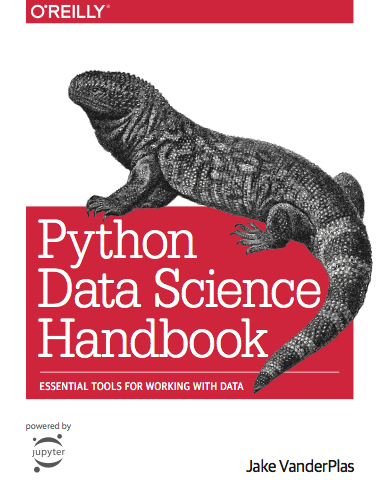## 元組( tuple )、列表( list )、字典( dictionary ) 儲存資料三兄弟

```test_1=5
test_2=2.3
test_3='apple'
print(type(test_1))
print(type(test_2))
print(type(test_3))```

1.元組tuple：

`tuple`有什麼特性呢?他有點像是那種刻在石板上的戒律。`tuple`一旦儲存起來，就無法做更動了，所以適合儲存那些不想要變動的值。以免不小心更改到值。

```x = (1, 'apple', 2, 'banana')
print(type(x))
print(x)```

2.列表list：

`list`的操作在 Python 中算是最常見也是最基礎的方式。簡單來說，他就是把一連串的資料，不管青紅皂白的儲存起來。比較需要注意的地方，在於你叫呼叫出`list`中的值，要搞清楚他的索引值。未來我會寫一篇關於操作`list`更詳細的介紹。

```y = [1, 'apple', 2, 'banana']
print(type(y))
print(y)```

3.字典dictionary：

`dictionary`的儲存方式，大家可以想像成英文單字卡本，也就是一面可以寫中文，一面可以寫英文的那種本子。他多了一個”鍵值”，他會跟你的資料做綁定，也就是你可以藉由鍵值來呼叫他所代表的資料。

`dictionary`的儲存方式利用”{}”，在並且一個鍵值和資料用”:”分隔，每一組使用”,”分隔。使用的方式如下

```z = {"apple":"蘋果", "banana":"香蕉", "one":1}
print(type(z))
print(z["apple"])
print(z["banana"])
print(z["one"])```

## 使用 Python 讀取 csv 和 xlsx 檔

(這邊可以先下載後面會用到的兩個資料，City_Zhvi_AllHomesgdplev)

#### 1.確認檔案類型：

2.讀取檔案：

(這邊先說讀取資料路徑有兩種方式：第一種是把你要讀取的檔案跟你的程式碼放在同一個資料夾，程式就會自己去抓；第二種是跟電腦講說你要讀取的檔案存在那邊。一般來說我比較喜歡第一種作法，因為這樣比較好掌握檔案的位置，若是使用第二種方法，要讀取的檔案位置變更的話，程式碼也要跟著跟動。所以下面是使用第一種方法，不過我會在附註放入第二種作法，大家有興趣可以參考~)```import pandas as pd

```import pandas as pd

```import pandas as pd```import pandas as pd## 十五分鐘內安裝 anaconda 寫第一支 Python 程式

Anaconda 可以想像成 Python的懶人包，傳統自己安裝自己安裝 Python 的話，還要進行路徑設定，安裝需要用的 Package 等對初學者不是那麼友善的步驟，但是安裝 Anaconda 可以幫你做好這些事情，也避免日後衍生比較複雜的問題。

###### 4.第一支小程式

`print("Hello world")`

## Python 裡面的閃電俠 — NumPy & Pandas

Numpy，雖然他是 Python 裡面的套件，但是他披著 Python 的皮，裡面的運作架構卻是使用 C 語言。而 Pandas 是利用 NumPy 的架構來表示或是紀錄更複雜的資料。哇，怎麼又扯出個”C語言”，你可能會開始懷該不會又要學新的東西了吧。NO! 這個就是 Python最爽的地方，其實你完全完全就可以不用管底下在幹嘛，你就可以利用這個套件，用Python 的語法，達成你要做的事情。不過為了讓大家更徹底的了解，我還是會跟大家講一下 Python 跟 C 語言的差別。下面的舉兩個例子來說明 Python 跟 C 語言運作上的差異(注意:這是舉例，不是完整的程式碼，所以不能拿來執行)

```int year, month, day;
year = 2018;
moth = 4;
day = 21;```

```year = 2018
month = 4
day = 21```

```float r, pi, area;
r = 3;
pi = 3.14;
area = (r ^2)*pi;```

```r = 3
pi = 3.14
area = (3^2)*pi```

## ( 免費 Python 電子書 ) Python Data Science Handbook ( Python 資料科學學習手冊)(2019/8/2 補充：想不到作者還有更新，最近發現他的程式碼整合到 colab 的當中，點選每個章節中的 Open in colab，便可以直接使用 colab 的環境來跑程式，當然也可以自己修試著程式，並測試看看!! )